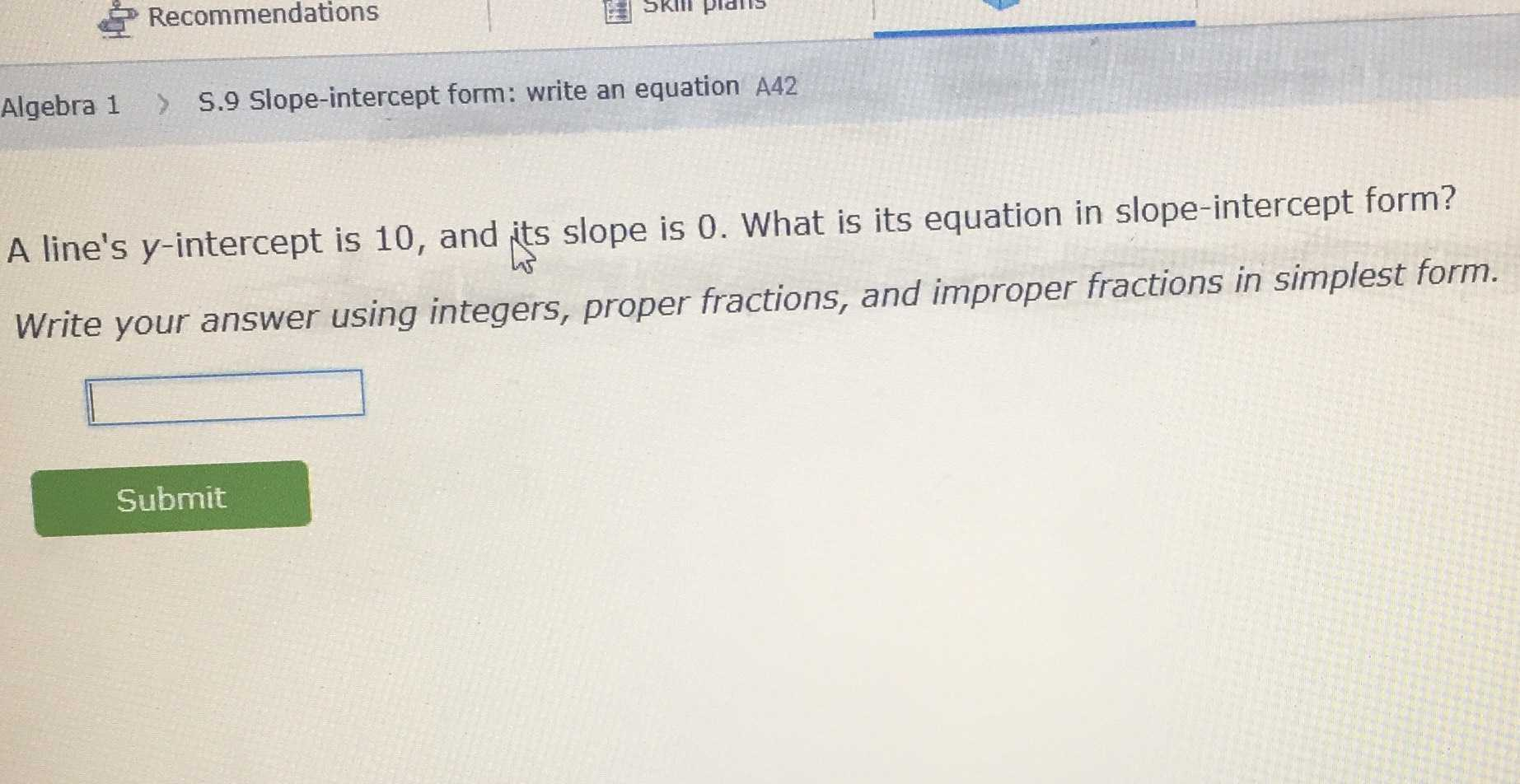### ¿Todavía tienes preguntas de matemáticas?

Pregunte a nuestros tutores expertos
Algebra
PreguntaA line's $$y$$ -intercept is 10 , and its slope is 0 . What is its equation in slope-intercept form?

Write your answer using integers, proper fractions, and improper fractions in simplest form.